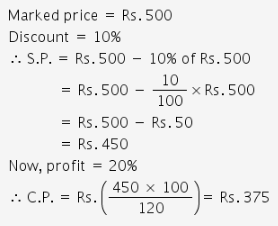Courses

# Profit And Loss MCQ

## 20 Questions MCQ Test Quantitative Techniques for CLAT | Profit And Loss MCQ

Description
This mock test of Profit And Loss MCQ for Quant helps you for every Quant entrance exam. This contains 20 Multiple Choice Questions for Quant Profit And Loss MCQ (mcq) to study with solutions a complete question bank. The solved questions answers in this Profit And Loss MCQ quiz give you a good mix of easy questions and tough questions. Quant students definitely take this Profit And Loss MCQ exercise for a better result in the exam. You can find other Profit And Loss MCQ extra questions, long questions & short questions for Quant on EduRev as well by searching above.
QUESTION: 1

Solution:
QUESTION: 2

Solution:
QUESTION: 3

### The marked price of a book is Rs. 160 and it is sold for Rs. 136. What was the rate of discount.

Solution:
QUESTION: 4

A man buys an article for Rs. 300 and sells it for Rs. 900. Find his gain per cent

Solution:
QUESTION: 5

A man buys a toy for Rs. 50 and sells it for Rs. 80. What is his gain per cent.

Solution:
QUESTION: 6

A man buys a bicycle for Rs. 450 and sells it for Rs. 300. Find his loss per cent.

Solution:
QUESTION: 7

A shopkeeper marks a saree 20% above the cost price and allows a discount to the purchases of 10%. What profit percent does he make.

Solution:
QUESTION: 8

By selling an article for Rs. 480 a person lost 20%. For how much should he sell it to make a profit of 20%.

Solution:
QUESTION: 9

The selling price of 5 article is the same as the cost price of 3 article. The gain or loss per cent is

Solution:
QUESTION: 10

A book seller bought 200 text book for Rs. 12000. He wanted to sell them at a profit so that he is left with 20 books as free. At what profit percent he sold them?

Solution:
According to question,
CP of 200 book = 12000
CP of 1 book =  12000 / 200
= Rs. 60
To get 20 books free
Profit = 20*60 = 1200
i.e. 10% of Rs. 12000
⇒10% gain
QUESTION: 11

A merchant finds that the CP of 2750 coconuts to be the same as the selling price of 2500 coconuts. Find the percent gain or loss.

Solution:
QUESTION: 12

A fair price shopkeeper taken 10% profit on his goods. He lost 20% goods during theft. His loss per cent is

Solution:
QUESTION: 13

A man sold 18 cots for Rs. 16800, gaining there by the cost price of 3 cots. Find the cost price of a cot.

Solution: Man sold 18 cots at 16800 and gained cost of 3 cots.

So we can assume total 21 cots. (18+3gained)
Price of 1cot =16800/21= 800rs
QUESTION: 14

Raghu purchased a scooter at 13/15th of its selling price and sold it for 12% more than the selling price. His gain is

Solution: CP=13 /15 SP
CP/SP =13/15

12%increase in SP =
15+15x12/100= 16.8

Profit =16.8 - 13 =3.8
Profit% = 3.8x100/13 = 29. 3/13 %
QUESTION: 15

The marked price of a pen is Rs. 60. After allowing a discount of 10% the merchant makes a profit of 20% what is the cost price of the pen.

Solution:
QUESTION: 16

A shopkeeper offers 10% discount on an article and still makes a profit of 20% what is the cost price of the article marked at Rs. 500?

Solution:QUESTION: 17

A dealer marked his goods 25% above the CP, and allows a 10% discount to his customers. What is his gain per cent.

Solution:
QUESTION: 18

The cost of manufacture of an articles is Rs. 900. Trader wants to gain 25% after giving a discount of 10%. The marked price should be

Solution:
QUESTION: 19

A shopkeeper purchases 12 baloons for Rs. 10 and sells them at 10 baloons for Rs. 12. He earns a profit of

Solution:
QUESTION: 20

Anu sold 2 books at Rs. 1.40 each. Her profit on one was 20% and her loss on the other was 20%. Then she made

Solution: By apply percentage effect. 20% - 20% - 20×20/100 = 4% loss occur. S.P of both books= 2.8 Rs = 100 - 4 = 96% of C.P. So, 2.8 = 96% , C.P 100% = 2.8×100/96 = 2.917. C.P - S.P = 2.917 - 2.8 = .117 = 12 paise